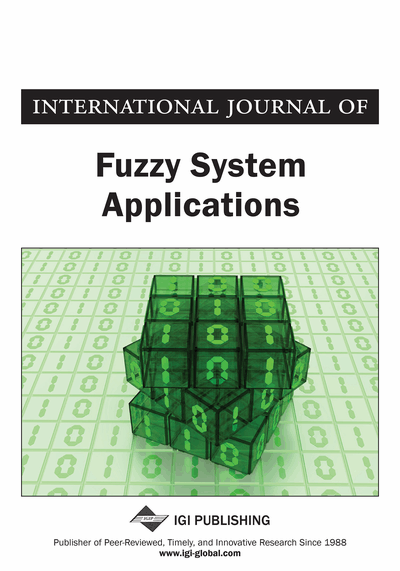# Fuzzy Continuity of Almost Linear Operators

Mark Burgin
DOI: 10.4018/ijfsa.2013010103
OnDemand:
(Individual Articles)
Available
\$37.50
No Current Special Offers

## Abstract

In this paper, the author studies relations between fuzzy continuity and boundedness of approximately linear operators in the context of neoclassical analysis. The main result of this paper (Theorem 1) demonstrates that for approximately linear operators, fuzzy continuity is equivalent to boundedness when the continuity defect (or measure of discontinuity) is sufficiently small. The classical result that describes continuity of linear operators becomes a direct corollary of this theorem. Applying Theorem 1, we demonstrate (Theorem 2) that for linear operators in normed vector spaces, fuzzy continuity coincides with continuity when the continuity defect is sufficiently small, i.e., when it is less than one. Results are oriented at applications in physics, theory of information and other fields where operator equations play an important role. Several open problems and directions for future research are considered at the end of the paper.
Article Preview
Top

## Introduction

Linear operators play an important role in physics (cf., for example, (von Neumann, 1996; Davies & Lewis, 1970; Kato, 1980; Byron & Fuller, 1992; Carfi, 2004; Dosi, 2011)), as well as in functional analysis (cf., for example, (Dunford & Schwartz, 1958; Rudin, 1991; Kolmogorov & Fomin, 1999)). An essential property of operators is continuity. One of the central results of functional analysis is the theorem that establishes equivalence between continuity and boundedness for linear operators.

Here we study these properties in the context of neoclassical analysis (Burgin, 2008). Neoclassical analysis extends methods of classical calculus to reflect uncertainties that arise in computation and measurements. In it, basic structures of the classical analysis, that is, operators, sequences, series, and operators, are studied by means of fuzzy concepts: fuzzy limits, fuzzy continuity, and fuzzy derivatives. For instance, continuous operators studied in the classical analysis become a part of the set of the fuzzy continuous operators studied in neoclassical analysis.

Aiming at representation of uncertainties and imprecision and extending the scope of the classical calculus and analysis, neoclassical analysis makes, at the same time, methods of the classical calculus more precise with respect to the real life applications. In particular, conditions on continuity are relaxed bringing researchers to fuzzy continuity and making calculus more adequate to scientific exploration where experimental data do not allow establishing continuity of functions and operators in the classical sense. Consequently, new results are obtained extending and even completing classical theorems. In addition, resources of analytical methods for various applications also become more broad and efficient.

In this paper, we consider operators that may be not exactly linear but approximately or almost linear. Mathematicians already studied problems in which morphisms are approximate homomorphisms in a given category. For instance, in the category of groups, one can consider mappings f: GH, where G and H are groups, such that f(xy) is not necessarily equal to f(x)f(y), but must be “close” in some sense to f(x)f(y) (Anderson, 1986). For abelian groups, an approximate homomorphism f has to satisfy a similar condition: f(x + y) is “close” in some sense to f(x) + f(y). A natural description of such closeness was suggested by Zlatoš and Špakula (2004). Taking two groups G and H with a (left) invariant metric ρ and a positive real number k, they define a k-homomorphism as a mapping f: GH for which ρ(f(x)f(y), f(xy)) ≤ k is true for all x, yG. In the case of normed vector spaces, an approximate homomorphism becomes an approximately linear mapping defined in this paper.

The main result of this paper (Theorem 1) demonstrates that for approximately linear operators, fuzzy continuity is equivalent to boundedness when the continuity defect (or measure of discontinuity) is sufficiently small. The classical result that describes continuity of linear operators becomes a direct corollary of this theorem. Applying Theorem 1 to linear operators in vector spaces, we demonstrate (Theorem 2) that in this case, fuzzy continuity coincides with continuity when the continuity defect is sufficiently small, i.e., when it is less than one.

## Complete Article List

Search this Journal:
Reset
Volume 13: 1 Issue (2024)
Volume 12: 1 Issue (2023)
Volume 11: 4 Issues (2022)
Volume 10: 4 Issues (2021)
Volume 9: 4 Issues (2020)
Volume 8: 4 Issues (2019)
Volume 7: 4 Issues (2018)
Volume 6: 4 Issues (2017)
Volume 5: 4 Issues (2016)
Volume 4: 4 Issues (2015)
Volume 3: 4 Issues (2013)
Volume 2: 4 Issues (2012)
Volume 1: 4 Issues (2011)
View Complete Journal Contents Listing